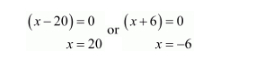# If two pipes function simultaneously, a reservoir will be filled in 12 hours.

Question:

If two pipes function simultaneously, a reservoir will be filled in 12 hours. One pipe fills the reservoir 10 hours faster than the other. How many hours will the second pipe take to fill the reservoir?

Solution:

Let the first pipe takes $x$ hours to fill the reservoir. Then the second pipe will takes $=(x+10)$ hours to fill the reservoir.

Since, the faster pipe takes $x$ hours to fill the reservoir.

Therefore, portion of the reservoir filled by the faster pipe in one hour $=\frac{1}{x}$

So, portion of the reservoir filled by the faster pipe in 12 hours $=\frac{12}{x}$

Similarly,

Portion of the reservoir filled by the slower pipe in 12 hours $=\frac{12}{x+10}$

It is given that the reservoir is filled in 12 hours.

So,

$\frac{12}{x}+\frac{12}{x+10}=1$

$\frac{12(x+10)+12 x}{x(x+10)}=1$

$12 x+120+12 x=x^{2}+10 x$

$x^{2}+10 x-24 x-120=0$

$x^{2}-14 x-120=0$

$x^{2}-20 x+6 x-120=0$

$x(x-20)+6(x-20)=0$

$(x-20)(x+6)=0$But, $x$ cannot be negative.

Therefore, when $x=20$ then

$(x+10)=20+10$

$=30$

Hence, the second pipe will takes 30 hours to fill the reservoir.